SSC CGL Previous Year Questions: Data Interpretation - 7

# SSC CGL Previous Year Questions: Data Interpretation - 7 - SSC CGL

Test Description

## 18 Questions MCQ Test SSC CGL (Tier - 1) - Previous Year Papers (Topic Wise) - SSC CGL Previous Year Questions: Data Interpretation - 7

SSC CGL Previous Year Questions: Data Interpretation - 7 for SSC CGL 2023 is part of SSC CGL (Tier - 1) - Previous Year Papers (Topic Wise) preparation. The SSC CGL Previous Year Questions: Data Interpretation - 7 questions and answers have been prepared according to the SSC CGL exam syllabus.The SSC CGL Previous Year Questions: Data Interpretation - 7 MCQs are made for SSC CGL 2023 Exam. Find important definitions, questions, notes, meanings, examples, exercises, MCQs and online tests for SSC CGL Previous Year Questions: Data Interpretation - 7 below.
Solutions of SSC CGL Previous Year Questions: Data Interpretation - 7 questions in English are available as part of our SSC CGL (Tier - 1) - Previous Year Papers (Topic Wise) for SSC CGL & SSC CGL Previous Year Questions: Data Interpretation - 7 solutions in Hindi for SSC CGL (Tier - 1) - Previous Year Papers (Topic Wise) course. Download more important topics, notes, lectures and mock test series for SSC CGL Exam by signing up for free. Attempt SSC CGL Previous Year Questions: Data Interpretation - 7 | 18 questions in 18 minutes | Mock test for SSC CGL preparation | Free important questions MCQ to study SSC CGL (Tier - 1) - Previous Year Papers (Topic Wise) for SSC CGL Exam | Download free PDF with solutions
 1 Crore+ students have signed up on EduRev. Have you?
SSC CGL Previous Year Questions: Data Interpretation - 7 - Question 1

### DIRECTIONS: The pie-chart given below shows the distribution of workforce by occupational category for country X in 1981 and 1995. Study the chart and answer the questions no. 15 to 19.       (SSC Sub. Ins. 2012)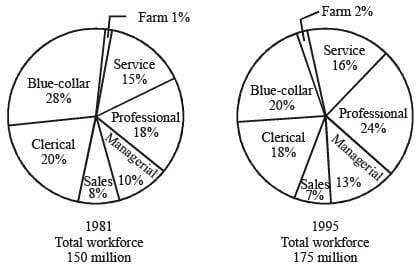Q. The increase in the number of Clerical workers in the workforce of country X from 1981 to 1995 (in millions) is:

Detailed Solution for SSC CGL Previous Year Questions: Data Interpretation - 7 - Question 1

Clerical % in country X in 1981 = 20% of 150 = 30
Clerical % in country X in 1995 = 18% of 175 = 31.5
So, increase = 1.5 million

SSC CGL Previous Year Questions: Data Interpretation - 7 - Question 2

### DIRECTIONS: The pie-chart given below shows the distribution of workforce by occupational category for country X in 1981 and 1995. Study the chart and answer the questions no. 15 to 19.       (SSC Sub. Ins. 2012)Q. The ratio of the number of workers in the Professional category in 1981 to the number of such workers in 1995 is

Detailed Solution for SSC CGL Previous Year Questions: Data Interpretation - 7 - Question 2

Ratio of workers to professional in 1981 to 1995
Professional in 1981 → 18%
⇒ 18% of 150 = 27
Professional in 1995 → 24%
⇒ 24% of 175 = 42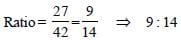SSC CGL Previous Year Questions: Data Interpretation - 7 - Question 3

### DIRECTIONS: The pie-chart given below shows the distribution of workforce by occupational category for country X in 1981 and 1995. Study the chart and answer the questions no. 15 to 19.       (SSC Sub. Ins. 2012)Q. In 1981, the number of categories which comprised of more than 25 million workers each, is

Detailed Solution for SSC CGL Previous Year Questions: Data Interpretation - 7 - Question 3

In 1981, no. of categories more than 25 million workers i.e. more than 16% of 150.
More than 16% is → Professional, clerical, Blue collar i.e. 3.

SSC CGL Previous Year Questions: Data Interpretation - 7 - Question 4

DIRECTIONS: The pie-chart given below shows the distribution of workforce by occupational category for country X in 1981 and 1995. Study the chart and answer the questions no. 15 to 19.       (SSC Sub. Ins. 2012)Q. In 1981, the number of Sevice workers in the workforce, in millions, was

Detailed Solution for SSC CGL Previous Year Questions: Data Interpretation - 7 - Question 4

In 1981, no. of service workers = 15% of 150 = 22.5 million

SSC CGL Previous Year Questions: Data Interpretation - 7 - Question 5

DIRECTIONS: The following graph shows the production of cotton bales of 100 kg each in lakhs by different states A, B, C, D and E over the years. Study the graph and answer Question Nos. 11 to 14.       (SSC CGL 2nd Sit.2011)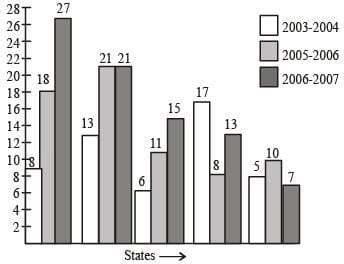Q. The number of States for which the production of cotton in 2005–2006 is less than or equal to the preceding year is

Detailed Solution for SSC CGL Previous Year Questions: Data Interpretation - 7 - Question 5

Only one state is production of cotton in (2005 – 2006) is less than the previous year.

SSC CGL Previous Year Questions: Data Interpretation - 7 - Question 6

DIRECTIONS: The following graph shows the production of cotton bales of 100 kg each in lakhs by different states A, B, C, D and E over the years. Study the graph and answer Question Nos. 11 to 14.       (SSC CGL 2nd Sit.2011)Q. How many kg of cotton was produced by State C durig the given period?

Detailed Solution for SSC CGL Previous Year Questions: Data Interpretation - 7 - Question 6

Total cotton production in state C = (6 + 11 + 15) lac × 100 kg = 320000000 kg

SSC CGL Previous Year Questions: Data Interpretation - 7 - Question 7

DIRECTIONS: The following graph shows the production of cotton bales of 100 kg each in lakhs by different states A, B, C, D and E over the years. Study the graph and answer Question Nos. 11 to 14.       (SSC CGL 2nd Sit.2011)Q. In which State(s) is there a steady increase in the production of cotton during the given period?

Detailed Solution for SSC CGL Previous Year Questions: Data Interpretation - 7 - Question 7

It is obvious from the graph.

SSC CGL Previous Year Questions: Data Interpretation - 7 - Question 8

DIRECTIONS: The following graph shows the production of cotton bales of 100 kg each in lakhs by different states A, B, C, D and E over the years. Study the graph and answer Question Nos. 11 to 14.       (SSC CGL 2nd Sit.2011)Q. The production of State C in 2003–2004 is how many times its production in 2006–2007?

Detailed Solution for SSC CGL Previous Year Questions: Data Interpretation - 7 - Question 8

Required answer = 6/15 = 0.4

SSC CGL Previous Year Questions: Data Interpretation - 7 - Question 9

DIRECTIONS: The following graph shows the demand and production of cotton by 5 companies A, B, C, D and E. Study the graph and answer questions.        (SSC CGL 1st Sit.2011)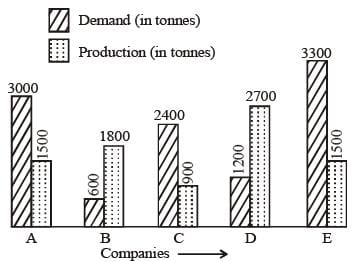Q. The demand for company B is what percent of the demand for company C?

Detailed Solution for SSC CGL Previous Year Questions: Data Interpretation - 7 - Question 9

Required percentage = (600/2400) x 100 = 25%

SSC CGL Previous Year Questions: Data Interpretation - 7 - Question 10

DIRECTIONS: The following graph shows the demand and production of cotton by 5 companies A, B, C, D and E. Study the graph and answer questions.        (SSC CGL 1st Sit.2011)Q. The production of company D is how many times that of the production of the company A?

Detailed Solution for SSC CGL Previous Year Questions: Data Interpretation - 7 - Question 10

Requried value = 2700/1500 = 1.8

SSC CGL Previous Year Questions: Data Interpretation - 7 - Question 11

DIRECTIONS: The following graph shows the demand and production of cotton by 5 companies A, B, C, D and E. Study the graph and answer questions.        (SSC CGL 1st Sit.2011)Q. What is the difference (in tonnes) between average demand and average production of the five companies taken together?

Detailed Solution for SSC CGL Previous Year Questions: Data Interpretation - 7 - Question 11

Required difference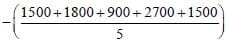= 2100 – 1680 = 420 tonnes

SSC CGL Previous Year Questions: Data Interpretation - 7 - Question 12

DIRECTIONS: The following graph shows the demand and production of cotton by 5 companies A, B, C, D and E. Study the graph and answer questions.        (SSC CGL 1st Sit.2011)Q. What is the ratio of companies having more demand than production to those having more production than demand?

Detailed Solution for SSC CGL Previous Year Questions: Data Interpretation - 7 - Question 12

Requried ratio = 3 : 2

SSC CGL Previous Year Questions: Data Interpretation - 7 - Question 13

DIRECTIONS: The pie chart, given here, shows the amount of money spent on various sports by a school administration in a particular year.
Observe the pie chart and answer the questions based on this graph.      (SSC CGL 2nd Sit.2010)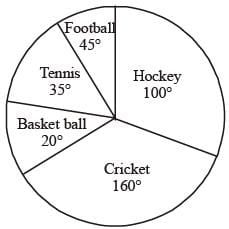Q. If the money spent on football is ₹ 9,000, then what was the total amount spent on all sports ?

Detailed Solution for SSC CGL Previous Year Questions: Data Interpretation - 7 - Question 13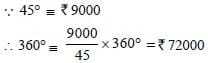SSC CGL Previous Year Questions: Data Interpretation - 7 - Question 14

DIRECTIONS: The pie chart, given here, shows the amount of money spent on various sports by a school administration in a particular year.
Observe the pie chart and answer the questions based on this graph.      (SSC CGL 2nd Sit.2010)Q. If the money spent on football was ₹9,000, what amount was spent on Cricket ?

Detailed Solution for SSC CGL Previous Year Questions: Data Interpretation - 7 - Question 14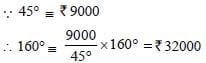SSC CGL Previous Year Questions: Data Interpretation - 7 - Question 15

DIRECTIONS: The pie chart, given here, shows the amount of money spent on various sports by a school administration in a particular year.
Observe the pie chart and answer the questions based on this graph.      (SSC CGL 2nd Sit.2010)Q. If the money spent on football was ₹ 9,000 how much more money was spent on hockey than on football?

Detailed Solution for SSC CGL Previous Year Questions: Data Interpretation - 7 - Question 15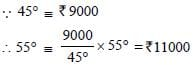SSC CGL Previous Year Questions: Data Interpretation - 7 - Question 16

DIRECTIONS: The pie chart, given here, represents the number of valid votes obtained by four students who contested election for school leadership. The total number of valid votes polled was 720.
Observe the chart and answer the questions based on it.       (SSC CGL 1st Sit. 2010)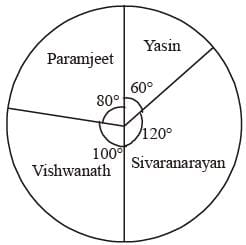Q. By how many votes did the winner defeat his nearest rival ?

Detailed Solution for SSC CGL Previous Year Questions: Data Interpretation - 7 - Question 16

Angles of the difference of votes of the winner and the nearest rival = 120 – 100 = 20°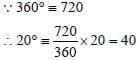SSC CGL Previous Year Questions: Data Interpretation - 7 - Question 17

DIRECTIONS: The pie chart, given here, represents the number of valid votes obtained by four students who contested election for school leadership. The total number of valid votes polled was 720.
Observe the chart and answer the questions based on it.       (SSC CGL 1st Sit. 2010)Q. Who was the winner....?

Detailed Solution for SSC CGL Previous Year Questions: Data Interpretation - 7 - Question 17

Sivaraman got the maximum votes. i.e.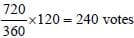He was the winner.

SSC CGL Previous Year Questions: Data Interpretation - 7 - Question 18

DIRECTIONS: The pie chart, given here, represents the number of valid votes obtained by four students who contested election for school leadership. The total number of valid votes polled was 720.
Observe the chart and answer the questions based on it.       (SSC CGL 1st Sit. 2010)Q. What was the minimum number of votes obtained by any candidate?

Detailed Solution for SSC CGL Previous Year Questions: Data Interpretation - 7 - Question 18

∵ 360° ≡ 720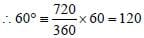## SSC CGL (Tier - 1) - Previous Year Papers (Topic Wise)

250 tests
Information about SSC CGL Previous Year Questions: Data Interpretation - 7 Page
In this test you can find the Exam questions for SSC CGL Previous Year Questions: Data Interpretation - 7 solved & explained in the simplest way possible. Besides giving Questions and answers for SSC CGL Previous Year Questions: Data Interpretation - 7, EduRev gives you an ample number of Online tests for practice

250 tests